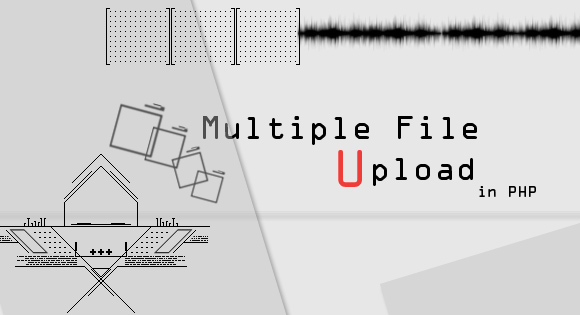# 如何使用PHP和MySQL進行多文件上傳

1 月 22, 2019 | | 0 條留言## HTML

``````<form action="" enctype="multipart/form-data" method="POST">
<input multiple="" name="files[]" type="file"></input>
<input type="submit/"></input>
</form>``````

## PHP

\$_FILES[\’ \’] 將包含每個文件的信息，但是當選擇多個文件時，它將包含在另一個數組中的每個文件的詳細信息。

``````foreach(\$_FILES['files']['tmp_name'] as \$key => \$tmp_name ){
\$file_name = \$key.\$_FILES['files']['name'][\$key];
\$file_size =\$_FILES['files']['size'][\$key];
\$file_tmp =\$_FILES['files']['tmp_name'][\$key];
\$file_type=\$_FILES['files']['type'][\$key];
}``````

``\$extensions = array(jpeg,jpg,png);   ``

``````\$file_ext=explode('.',\$_FILES['image']['name'][\$key])   ;
\$file_ext=end(\$file_ext);
\$file_ext=strtolower(end(explode('.',\$_FILES['image']['name'][\$key])));
if(in_array(\$file_ext,\$extensions ) === false){
\$errors[]=extension not allowed;
}     ``````

``````if(\$_FILES['image']['size'][\$key] > 2097152){
\$errors[]='File size must be less tham 2 MB';
}   ``````

## SQL

``````CREATE TABLE `upload_data` (
`ID` int(5) NOT NULL AUTO_INCREMENT,
`USER_CODE` int(4) unsigned zerofill NOT NULL,
`FILE_NAME` varchar(200) NOT NULL,
`FILE_SIZE` varchar(200) NOT NULL,
`FILE_TYPE` varchar(200) NOT NULL,
PRIMARY KEY (`ID`)
)``````

USER_CODE 如果您有基於用戶的應用程序或任何可識別每個項目的唯一ID，則每個用戶都是唯一的。

``````\$query=INSERT into upload_data (`EMP_CODE`,`FILE_NAME`,`FILE_SIZE`,`FILE_TYPE`) VALUES('\$codes','\$file_name','\$file_size','\$file_type'); ;
# Make sure that the query is not executed unless the \$errors array is empty.
mysql_query(\$query);``````

## 移動文件

``````if(is_dir(\$dir)==false){
}``````

## 重命名文件

OLD_FILE_PATH文件名，包含擴展名和位置以及 NEW_FILE_NAME新名稱。

``````#Check if a file of same name exist
if(is_dir(emp_data/\$codes/.\$file_name)==false){
}else{
rename(\$file_tmp, \$desired_dir/.\$file_name);
# rename can move the file along with renaming it
}``````

## 曲折

``````<?php if(isset(\$_FILES['files'])){
\$errors= array();
foreach(\$_FILES['files']['tmp_name'] as \$key =?> \$tmp_name ){
\$file_name = \$key.\$_FILES['files']['name'][\$key];
\$file_size =\$_FILES['files']['size'][\$key];
\$file_tmp =\$_FILES['files']['tmp_name'][\$key];
\$file_type=\$_FILES['files']['type'][\$key];
if(\$file_size > 2097152){
\$errors[]='File size must be less than 2 MB';
}
\$query=INSERT into upload_data (`USER_ID`,`FILE_NAME`,`FILE_SIZE`,`FILE_TYPE`) VALUES('\$user_id','\$file_name','\$file_size','\$file_type'); ;
\$desired_dir=user_data;
if(empty(\$errors)==true){
if(is_dir(\$desired_dir)==false){
mkdir(\$desired_dir, 0700);        // Create directory if it does not exist
}
if(is_dir(\$desired_dir/.\$file_name)==false){
}else{
//rename the file if another one exist
\$new_dir=user_data/.\$file_name.time();
rename(\$file_tmp,\$new_dir) ;
}
mysql_query(\$query);
}else{
print_r(\$errors);
}
}
if(empty(\$error)){
echo Success;
}
}
?>
<form action="" enctype="multipart/form-data" method="POST">
<input multiple="" name="files[]" type="file"></input>
<input type="submit/"></input>
</form>``````